ASE Tweeter Full range Mids Woofer Subwoofer Car-HiFi no longer available
 Illuminator Revelator Classic Discovery

## ScanSpeak

Founded in 1970, Scan-Speak still resides at its original address in Videbaek, Denmark. Located in the heart of the Jutland peninsula, the acoustic engineers adhere to the legendary policy of "Never compromise".

## Illuminatoropen a bigger photo

D2004/602000       order no. ss-D2004-6020       EUR 115,02excl. VAT: € 96.66 / \$ 109.22
19 mm tweeter with fabric cone.
• power handling (continuous/programme) = 50/120 W
• frequency range = 1000-35000 Hz
• resonance frequency fs = 600 Hz
• impedance R = 4 Ohm
• sound pressure level SPL = 88,4 dB (2,83V; 1m)
• DC resistance Re = 2,8 Ohm
• force factor BL = 1,3 N/A
• voice coil inductance L = 0,02 mH
• effective piston radiating area Sd = 4,5 cm2
• effective mechanical mass incl. air load mms = 0,22 g
• equivalent volume of compliance Vas = 0,01 l
• total Q factor Qts = 1,07 (Qms=4,42, Qes=1,42)
• voice coil diameter = 19 mm
• maximum peak linear excursion vibration xlin = +/- 0,4 mm
• mounting diameter d = 42,1 mm
• overall diameter d = 55,3 mm
• mounting depth (not countersunk) t = 34,3 mm
PDF data sheet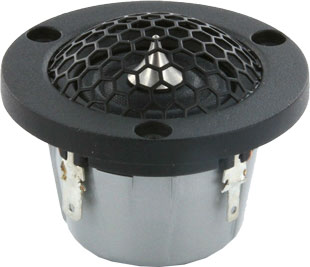open a bigger photo

R2004/602000       order no. ss-R2004-6020       EUR 143,47excl. VAT: € 120.56 / \$ 136.24
19 mm tweeter with fabric cone.
• power handling (continuous/programme) = 50 W
• frequency range = 1000-35000 Hz
• resonance frequency fs = 675 Hz
• impedance R = 4 Ohm
• sound pressure level SPL = 86 dB (2,83V; 1m)
• DC resistance Re = 2,8 Ohm
• force factor BL = 1,3 N/A
• voice coil inductance L = 0,02 mH
• effective piston radiating area Sd = 3,8 cm2
• effective mechanical mass incl. air load mms = 0,22 g
• equivalent volume of compliance Vas = 0,01 l
• total Q factor Qts = 1,01 (Qms=3,01, Qes=1,51)
• voice coil diameter = 19 mm
• maximum peak linear excursion vibration xlin = +/- 0,4 mm
• mounting diameter d = 42,1 mm
• overall diameter d = 55,3 mm
• mounting depth (not countersunk) t = 34,3 mm
PDF data sheet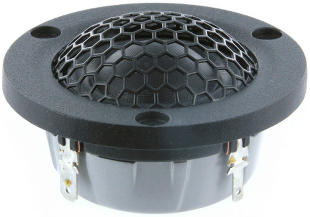open a bigger photo

D3004/602000       order no. ss-D3004-6020       EUR 114,99excl. VAT: € 96.63 / \$ 109.19
26 mm tweeter with fabric cone.
• power handling (continuous/programme) = 50/130 W
• frequency range = 1000-30000 Hz
• resonance frequency fs = 700 Hz
• impedance R = 4 Ohm
• sound pressure level SPL = 89,2 dB (2,83V; 1m)
• DC resistance Re = 3 Ohm
• force factor BL = 1,7 N/A
• voice coil inductance L = 0,02 mH
• effective piston radiating area Sd = 7 cm2
• effective mechanical mass incl. air load mms = 0,35 g
• equivalent volume of compliance Vas = 0,01 l
• total Q factor Qts = 1,15 (Qms=4,15, Qes=1,6)
• voice coil diameter = 26 mm
• maximum peak linear excursion vibration xlin = +/- 0,2 mm
• mounting diameter d = 48,5 mm
• overall diameter d = 61,9 mm
• mounting depth (not countersunk) t = 26,5 mm
PDF data sheetopen a bigger photo

R3004/602000       order no. ss-R3004-6020       EUR 148,70excl. VAT: € 124.96 / \$ 141.20
26 mm tweeter with fabric cone.
• power handling (continuous/programme) = 50/130 W
• frequency range = 1000-30000 Hz
• resonance frequency fs = 625 Hz
• impedance R = 4 Ohm
• sound pressure level SPL = 87,4 dB (2,83V; 1m)
• DC resistance Re = 3 Ohm
• force factor BL = 1,7 N/A
• voice coil inductance L = 0,02 mH
• effective piston radiating area Sd = 5,6 cm2
• effective mechanical mass incl. air load mms = 0,35 g
• equivalent volume of compliance Vas = 0,01 l
• total Q factor Qts = 1,14 (Qms=5,73, Qes=1,43)
• voice coil diameter = 26 mm
• maximum peak linear excursion vibration xlin = +/- 0,2 mm
• mounting diameter d = 48,4 mm
• overall diameter d = 61,9 mm
• mounting depth (not countersunk) t = 26,5 mm
PDF data sheet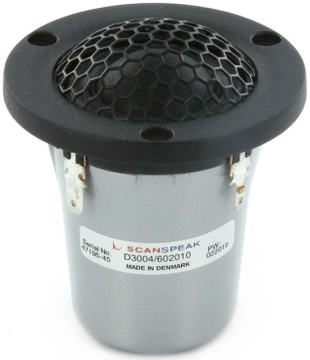open a bigger photo

D3004/602010       order no. ss-D3004-602010       EUR 125,43excl. VAT: € 105.40 / \$ 119.11
26 mm tweeter with fabric cone.
• power handling (continuous/programme) = 50/130 W
• frequency range = 1000-30000 Hz
• resonance frequency fs = 425 Hz
• impedance R = 4 Ohm
• sound pressure level SPL = 89,6 dB (2,83V; 1m)
• DC resistance Re = 3 Ohm
• force factor BL = 1,7 N/A
• voice coil inductance L = 0,02 mH
• effective piston radiating area Sd = 7 cm2
• effective mechanical mass incl. air load mms = 0,35 g
• equivalent volume of compliance Vas = 0,03 l
• total Q factor Qts = 0,7 (Qms=2,5, Qes=0,97)
• voice coil diameter = 26 mm
• maximum peak linear excursion vibration xlin = +/- 0,2 mm
• mounting diameter d = 48,4 mm
• overall diameter d = 61,9 mm
• mounting depth (not countersunk) t = 60,4 mm
PDF data sheet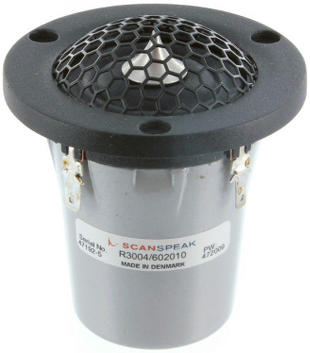open a bigger photo

R3004/602010       order no. ss-R3004-602010       EUR 156,80excl. VAT: € 131.76 / \$ 148.89
26 mm tweeter with fabric cone.
• power handling (continuous/programme) = 50/130 W
• frequency range = 1000-35000 Hz
• resonance frequency fs = 420 Hz
• impedance R = 4 Ohm
• sound pressure level SPL = 87,4 dB (2,83V; 1m)
• DC resistance Re = 3 Ohm
• force factor BL = 1,7 N/A
• voice coil inductance L = 0,02 mH
• effective piston radiating area Sd = 5,6 cm2
• effective mechanical mass incl. air load mms = 0,35 g
• equivalent volume of compliance Vas = 0,02 l
• total Q factor Qts = 0,74 (Qms=2,57, Qes=1,03)
• voice coil diameter = 26 mm
• maximum peak linear excursion vibration xlin = +/- 0,2 mm
• mounting diameter d = 48,4 mm
• overall diameter d = 61,9 mm
• mounting depth (not countersunk) t = 60,4 mm
PDF data sheet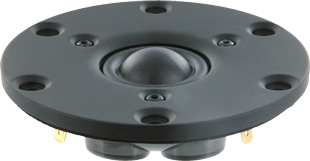open a bigger photo

recommended cabinet 1:
closed cabinet with 0,01 L volume
from 745/470 Hz (-3dB/-8dB)

recommended cabinet 2:
0,05 L volume bass reflex cabinet
with 2cm-Rohr reflex tube, 14 cm long.
from 343/291 Hz (-3dB/-8dB).

D3004/660000       order no. ss-D3004-6600       EUR 202,10excl. VAT: € 169.83 / \$ 191.91
26 mm dome tweeter with fabric dome.
• power handling (continuous/programme) = 90/150 W
• frequency range = 1000-35000 Hz
• resonance frequency fs = 470 Hz
• impedance R = 4 Ohm
• sound pressure level SPL = 91,5 dB (2,83V; 1m)
• DC resistance Re = 3 Ohm
• force factor BL = 2,3 N/A
• voice coil inductance L = 0,03 mH
• effective piston radiating area Sd = 7 cm2
• effective mechanical mass incl. air load mms = 0,35 g
• equivalent volume of compliance Vas = 0,02 l
• total Q factor Qts = 0,49 (Qms=3, Qes=0,59)
• voice coil diameter = 26 mm
• maximum peak linear excursion vibration xlin = +/- 0,2 mm
• mounting diameter d = 77 mm
• overall diameter d = 104 mm
• mounting depth (not countersunk) t = 28 mm
PDF data sheetopen a bigger photo

recommended cabinet 1:
closed cabinet with 0,02 L volume
from 723/456 Hz (-3dB/-8dB)

recommended cabinet 2:
0,06 L volume bass reflex cabinet
with 2cm-Rohr reflex tube, 10 cm long.
from 325/282 Hz (-3dB/-8dB).

D3004/662000       order no. ss-D3004-6620       EUR 219,54excl. VAT: € 184.49 / \$ 208.47
26 mm dome tweeter with fabric dome.
• power handling (continuous/programme) = 90/150 W
• frequency range = 1000-35000 Hz
• resonance frequency fs = 500 Hz
• impedance R = 4 Ohm
• sound pressure level SPL = 91,6 dB (2,83V; 1m)
• DC resistance Re = 3 Ohm
• force factor BL = 2,3 N/A
• voice coil inductance L = 0,03 mH
• effective piston radiating area Sd = 7 cm2
• effective mechanical mass incl. air load mms = 0,35 g
• equivalent volume of compliance Vas = 0,02 l
• total Q factor Qts = 0,53 (Qms=3,79, Qes=0,62)
• voice coil diameter = 26 mm
• maximum peak linear excursion vibration xlin = +/- 0,2 mm
• mounting diameter d = 82,7 mm
• overall diameter d = 104,25 mm
• mounting depth (not countersunk) t = 29 mm
PDF data sheet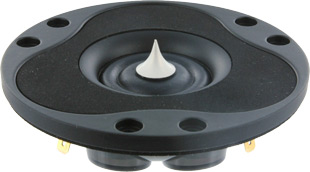open a bigger photo

recommended cabinet 1:
closed cabinet with 0,01 L volume
from 840/530 Hz (-3dB/-8dB)

recommended cabinet 2:
0,02 L volume bass reflex cabinet
with 2cm-Rohr reflex tube, 24 cm long.
from 389/328 Hz (-3dB/-8dB).

R3004/662000       order no. ss-R3004-6620       EUR 254,41excl. VAT: € 213.79 / \$ 241.58
26 mm tweeter with fabric cone.
• power handling (continuous/programme) = 90/150 W
• frequency range = 1000-35000 Hz
• resonance frequency fs = 520 Hz
• impedance R = 4 Ohm
• sound pressure level SPL = 90,4 dB (2,83V; 1m)
• DC resistance Re = 3 Ohm
• force factor BL = 2,3 N/A
• voice coil inductance L = 0,03 mH
• effective piston radiating area Sd = 5,6 cm2
• effective mechanical mass incl. air load mms = 0,3 g
• equivalent volume of compliance Vas = 0,01 l
• total Q factor Qts = 0,48 (Qms=3,27, Qes=0,56)
• voice coil diameter = 26 mm
• maximum peak linear excursion vibration xlin = +/- 0,2 mm
• mounting diameter d = 82,7 mm
• overall diameter d = 104,25 mm
• mounting depth (not countersunk) t = 29 mm
PDF data sheetopen a bigger photo

recommended cabinet 1:
closed cabinet with 0,02 L volume
from 723/456 Hz (-3dB/-8dB)

recommended cabinet 2:
0,06 L volume bass reflex cabinet
with 2cm-Rohr reflex tube, 10 cm long.
from 325/282 Hz (-3dB/-8dB).

D3004/664000       order no. ss-D3004-6640       EUR 479,00excl. VAT: € 402.52 / \$ 454.85
26 mm dome tweeter with beryllium dome.
• power handling (continuous/programme) = 90/150 W
• frequency range = 1000-40000 Hz
• resonance frequency fs = 500 Hz
• impedance R = 4 Ohm
• sound pressure level SPL = 91,5 dB (2,83V; 1m)
• DC resistance Re = 3 Ohm
• force factor BL = 2,3 N/A
• voice coil inductance L = 0,03 mH
• effective piston radiating area Sd = 7 cm2
• effective mechanical mass incl. air load mms = 0,35 g
• equivalent volume of compliance Vas = 0,02 l
• total Q factor Qts = 0,53 (Qms=3,79, Qes=0,62)
• voice coil diameter = 26 mm
• maximum peak linear excursion vibration xlin = +/- 0,2 mm
• mounting diameter d = 77 mm
• overall diameter d = 104 mm
• mounting depth (not countersunk) t = 28 mm
PDF data sheet

## Revelatorrecommended cabinet 1:
closed cabinet with 0 L volume
from 1190/751 Hz (-3dB/-8dB)

recommended cabinet 2:
0,01 L volume bass reflex cabinet
with 2cm-Rohr reflex tube, 40 cm long.
from 621/487 Hz (-3dB/-8dB).

R2904/700000       order no. ss-R2904-7000       EUR 322,94excl. VAT: € 271.38 / \$ 306.66
26 mm tweeter with fabric cone.
• power handling (continuous/programme) = 160 W
• frequency range = 1200-38000 Hz
• resonance frequency fs = 520 Hz
• impedance R = 4 Ohm
• sound pressure level SPL = 94,5 dB (2,83V; 1m)
• DC resistance Re = 3 Ohm
• force factor BL = 2,8 N/A
• voice coil inductance L = 0,01 mH
• effective piston radiating area Sd = 5,6 cm2
• effective mechanical mass incl. air load mms = 0,3 g
• equivalent volume of compliance Vas = 0,01 l
• total Q factor Qts = 0,34 (Qms=2,9, Qes=0,38)
• voice coil diameter = 26 mm
• maximum peak linear excursion vibration xlin = +/- 0,2 mm
• mounting diameter d = 70 mm
• overall diameter d = 104,2 mm
• mounting depth (not countersunk) t = 33,5 mm
PDF data sheetopen a bigger photo

recommended cabinet 1:
closed cabinet with 0 L volume
from 1190/751 Hz (-3dB/-8dB)

recommended cabinet 2:
0,01 L volume bass reflex cabinet
with 2cm-Rohr reflex tube, 40 cm long.
from 621/487 Hz (-3dB/-8dB).

R2904/700005       order no. ss-R2904-700005       EUR 323,00excl. VAT: € 271.43 / \$ 306.71
26 mm tweeter with fabric cone.
• power handling (continuous/programme) = 160 W
• frequency range = 1200-38000 Hz
• resonance frequency fs = 520 Hz
• impedance R = 4 Ohm
• sound pressure level SPL = 94,5 dB (2,83V; 1m)
• DC resistance Re = 3 Ohm
• force factor BL = 2,8 N/A
• voice coil inductance L = 0,01 mH
• effective piston radiating area Sd = 5,6 cm2
• effective mechanical mass incl. air load mms = 0,3 g
• equivalent volume of compliance Vas = 0,01 l
• total Q factor Qts = 0,34 (Qms=2,9, Qes=0,38)
• voice coil diameter = 26 mm
• maximum peak linear excursion vibration xlin = +/- 0,2 mm
• mounting diameter d = 70 mm
• overall diameter d = 104,2 mm
• mounting depth (not countersunk) t = 33,5 mm
PDF data sheet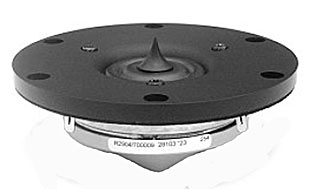recommended cabinet 1:
closed cabinet with 0 L volume
from 1190/751 Hz (-3dB/-8dB)

recommended cabinet 2:
0,01 L volume bass reflex cabinet
with 2cm-Rohr reflex tube, 40 cm long.
from 621/487 Hz (-3dB/-8dB).

R2904/700009       order no. ss-R2904-700009       EUR 324,10excl. VAT: € 272.35 / \$ 307.76
26 mm tweeter with fabric cone.
• power handling (continuous/programme) = 160 W
• frequency range = 1200-38000 Hz
• resonance frequency fs = 520 Hz
• impedance R = 4 Ohm
• sound pressure level SPL = 94,5 dB (2,83V; 1m)
• DC resistance Re = 3 Ohm
• force factor BL = 2,8 N/A
• voice coil inductance L = 0,01 mH
• effective piston radiating area Sd = 5,6 cm2
• effective mechanical mass incl. air load mms = 0,3 g
• equivalent volume of compliance Vas = 0,01 l
• total Q factor Qts = 0,34 (Qms=2,9, Qes=0,38)
• voice coil diameter = 26 mm
• maximum peak linear excursion vibration xlin = +/- 0,2 mm
• mounting diameter d = 70 mm
• overall diameter d = 104,2 mm
• mounting depth (not countersunk) t = 33,5 mm
PDF data sheetrecommended cabinet 1:
closed cabinet with 0,01 L volume
from 912/575 Hz (-3dB/-8dB)

recommended cabinet 2:
0,03 L volume bass reflex cabinet
with 2cm-Rohr reflex tube, 13 cm long.
from 433/358 Hz (-3dB/-8dB).

D2904/710002       order no. ss-D2904-710002       EUR 266,02excl. VAT: € 223.55 / \$ 252.61
26 mm dome tweeter with fabric dome.
• power handling (continuous/programme) = 90/150 W
• frequency range = 1000-30000 Hz
• resonance frequency fs = 520 Hz
• impedance R = 4 Ohm
• sound pressure level SPL = 94,4 dB (2,83V; 1m)
• DC resistance Re = 3 Ohm
• force factor BL = 2,8 N/A
• voice coil inductance L = 0,01 mH
• effective piston radiating area Sd = 7 cm2
• effective mechanical mass incl. air load mms = 0,4 g
• equivalent volume of compliance Vas = 0,02 l
• total Q factor Qts = 0,44 (Qms=3,5, Qes=0,5)
• voice coil diameter = 26 mm
• maximum peak linear excursion vibration xlin = +/- 0,2 mm
• mounting diameter d = 70 mm
• overall diameter d = 104,2 mm
• mounting depth (not countersunk) t = 33,9 mm
PDF data sheet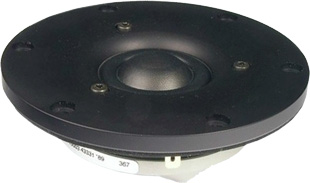open a bigger photo

recommended cabinet 1:
closed cabinet with 0,01 L volume
from 912/575 Hz (-3dB/-8dB)

recommended cabinet 2:
0,03 L volume bass reflex cabinet
with 2cm-Rohr reflex tube, 13 cm long.
from 433/358 Hz (-3dB/-8dB).

D2904/710003       order no. ss-D2904-710003       EUR 265,14excl. VAT: € 222.81 / \$ 251.77
26 mm dome tweeter with fabric dome.
• power handling (continuous/programme) = 90/150 W
• frequency range = 1000-30000 Hz
• resonance frequency fs = 520 Hz
• impedance R = 4 Ohm
• sound pressure level SPL = 94,4 dB (2,83V; 1m)
• DC resistance Re = 3 Ohm
• force factor BL = 2,8 N/A
• voice coil inductance L = 0,01 mH
• effective piston radiating area Sd = 7 cm2
• effective mechanical mass incl. air load mms = 0,4 g
• equivalent volume of compliance Vas = 0,02 l
• total Q factor Qts = 0,44 (Qms=3,5, Qes=0,5)
• voice coil diameter = 26 mm
• maximum peak linear excursion vibration xlin = +/- 0,2 mm
• mounting diameter d = 70 mm
• overall diameter d = 104,2 mm
• mounting depth (not countersunk) t = 33,9 mm
PDF data sheetopen a bigger photo

recommended cabinet 1:
closed cabinet with 0,01 L volume
from 917/579 Hz (-3dB/-8dB)

recommended cabinet 2:
0,03 L volume bass reflex cabinet
with HP35 reflex tube, 31 cm long.
from 433/360 Hz (-3dB/-8dB).

D2908/714000       order no. ss-D2908-714000       EUR 509,97excl. VAT: € 428.55 / \$ 484.26
26 mm dome tweeter with beryllium dome.
• power handling (continuous/programme) = 130/200 W
• frequency range = 1000-38000 Hz
• resonance frequency fs = 534 Hz
• impedance R = 8 Ohm
• sound pressure level SPL = 92 dB (2,83V; 1m)
• DC resistance Re = 5,7 Ohm
• force factor BL = 3,6 N/A
• voice coil inductance L = 0,02 mH
• effective piston radiating area Sd = 7 cm2
• effective mechanical mass incl. air load mms = 0,33 g
• equivalent volume of compliance Vas = 0,02 l
• total Q factor Qts = 0,47 (Qms=7,3, Qes=0,5)
• voice coil diameter = 26 mm
• maximum peak linear excursion vibration xlin = +/- 0,2 mm
• mounting diameter d = 90 mm
• overall diameter d = 120 mm
• mounting depth (not countersunk) t = 34,2 mm
PDF data sheetopen a bigger photo

D2905/990000       order no. ss-D2905-9900       EUR 228,84excl. VAT: € 192.30 / \$ 217.30
28 mm dome tweeter with fabric dome.
• power handling (continuous/programme) = 225/430 W
• frequency range = 900-30000 Hz
• resonance frequency fs = 500 Hz
• impedance R = 6 Ohm
• sound pressure level SPL = 91 dB (2,83V; 1m)
• DC resistance Re = 4,7 Ohm
• force factor BL = 3,5 N/A
• voice coil inductance L = 0,01 mH
• effective piston radiating area Sd = 8,5 cm2
• effective mechanical mass incl. air load mms = 0,45 g
• equivalent volume of compliance Vas = 0,02 l
• total Q factor Qts = 0,63 (Qms=4,83, Qes=0,72)
• voice coil diameter = 28 mm
• maximum peak linear excursion vibration xlin = +/- 0,4 mm
• mounting diameter d = 95 mm
• overall diameter d = 130 mm
• mounting depth (not countersunk) t = 54,2 mm
PDF data sheet

## Classic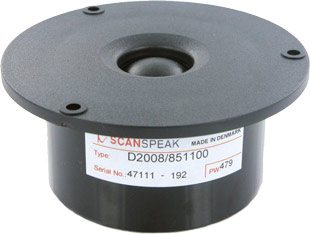open a bigger photo

recommended cabinet 1:
closed cabinet with 0,01 L volume
from 923/583 Hz (-3dB/-8dB)

recommended cabinet 2:
0,03 L volume bass reflex cabinet
with 2cm-Rohr reflex tube, 12 cm long.
from 413/360 Hz (-3dB/-8dB).

D2008/8511       order no. ss-D2008-8511       EUR 72,02excl. VAT: € 60.52 / \$ 68.39
19,4 mm dome tweeter with fabric dome.
• power handling (continuous/programme) = 90/150 W
• frequency range = 1500-21000 Hz
• resonance frequency fs = 650 Hz
• impedance R = 8 Ohm
• sound pressure level SPL = 89 dB (2,83V; 1m)
• DC resistance Re = 5,7 Ohm
• force factor BL = 2,4 N/A
• voice coil inductance L = 0,08 mH
• effective piston radiating area Sd = 3,8 cm2
• effective mechanical mass incl. air load mms = 0,2 g
• equivalent volume of compliance Vas = 0,01 l
• total Q factor Qts = 0,57 (Qms=1,9, Qes=0,81)
• voice coil diameter = 19,4 mm
• maximum peak linear excursion vibration xlin = +/- 0,8 mm
• mounting diameter d = 69 mm
• overall diameter d = 92 mm
• mounting depth (not countersunk) t = 41,1 mm
PDF data sheet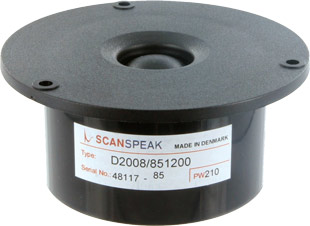open a bigger photo

D2008/8512       order no. ss-D2008-8512       EUR 72,05excl. VAT: € 60.55 / \$ 68.42
19 mm dome tweeter with fabric dome. The voice coil windings are immersed in magnetic fluid to increase short term power handling capacity, to reduce the compression and the Q factor..
• power handling (continuous/programme) = 90/150 W
• frequency range = 1500-21000 Hz
• resonance frequency fs = 800 Hz
• impedance R = 8 Ohm
• sound pressure level SPL = 88 dB (2,83V; 1m)
• DC resistance Re = 5,7 Ohm
• force factor BL = 2,4 N/A
• voice coil inductance L = 0,08 mH
• effective piston radiating area Sd = 3,8 cm2
• effective mechanical mass incl. air load mms = 0,25 g
• equivalent volume of compliance Vas = 0,01 l
• total Q factor Qts = 0,62 (Qms=1,26, Qes=1,24)
• voice coil diameter = 19 mm
• maximum peak linear excursion vibration xlin = +/- 0,7 mm
• mounting diameter d = 69 mm
• overall diameter d = 92 mm
• mounting depth (not countersunk) t = 41,1 mm
PDF data sheetopen a bigger photo

recommended cabinet 1:
closed cabinet with 0,01 L volume
from 923/583 Hz (-3dB/-8dB)

recommended cabinet 2:
0,03 L volume bass reflex cabinet
with 2cm-Rohr reflex tube, 12 cm long.
from 413/360 Hz (-3dB/-8dB).

D2010/8511       order no. ss-D2010-8511       EUR 72,13excl. VAT: € 60.61 / \$ 68.49
19,4 mm dome tweeter with fabric dome.
• power handling (continuous/programme) = 90/150 W
• frequency range = 1500-21000 Hz
• resonance frequency fs = 650 Hz
• impedance R = 8 Ohm
• sound pressure level SPL = 89 dB (2,83V; 1m)
• DC resistance Re = 5,7 Ohm
• force factor BL = 2,4 N/A
• voice coil inductance L = 0,08 mH
• effective piston radiating area Sd = 3,8 cm2
• effective mechanical mass incl. air load mms = 0,2 g
• equivalent volume of compliance Vas = 0,01 l
• total Q factor Qts = 0,57 (Qms=1,9, Qes=0,81)
• voice coil diameter = 19,4 mm
• maximum peak linear excursion vibration xlin = +/- 0,8 mm
• mounting diameter d = 69 mm
• overall diameter d = 98 mm
• mounting depth (not countersunk) t = 46 mm
PDF data sheet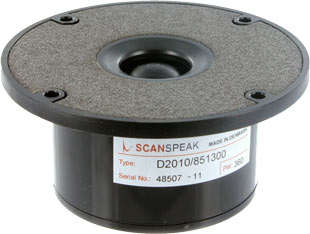open a bigger photo

D2010/8513       order no. ss-D2010-8513       EUR 79,03excl. VAT: € 66.41 / \$ 75.05
19 mm dome tweeter with fabric dome. The voice coil windings are immersed in magnetic fluid to increase short term power handling capacity, to reduce the compression and the Q factor..
• power handling (continuous/programme) = 90/150 W
• frequency range = 4000-20000 Hz
• resonance frequency fs = 800 Hz
• impedance R = 8 Ohm
• sound pressure level SPL = 88 dB (2,83V; 1m)
• DC resistance Re = 5,7 Ohm
• force factor BL = 2,4 N/A
• voice coil inductance L = 0,08 mH
• effective piston radiating area Sd = 3,8 cm2
• effective mechanical mass incl. air load mms = 0,25 g
• equivalent volume of compliance Vas = 0,01 l
• total Q factor Qts = 0,62 (Qms=1,26, Qes=1,24)
• voice coil diameter = 19 mm
• maximum peak linear excursion vibration xlin = +/- 0,7 mm
• mounting diameter d = 69 mm
• overall diameter d = 98 mm
• mounting depth (not countersunk) t = 46 mm
PDF data sheetopen a bigger photo

D2904/980000       order no. ss-D2904-9800       EUR 199,85excl. VAT: € 167.94 / \$ 189.77
28 mm dome tweeter with aluminium dome.
• power handling (continuous/programme) = 160 W
• frequency range = 1000-22000 Hz
• resonance frequency fs = 500 Hz
• impedance R = 4 Ohm
• sound pressure level SPL = 88 dB (2,83V; 1m)
• DC resistance Re = 3,5 Ohm
• force factor BL = 2,8 N/A
• voice coil inductance L = 0,01 mH
• effective piston radiating area Sd = 8,5 cm2
• effective mechanical mass incl. air load mms = 0,6 g
• equivalent volume of compliance Vas = 0,02 l
• total Q factor Qts = 0,66 (Qms=3,09, Qes=0,84)
• voice coil diameter = 28 mm
• maximum peak linear excursion vibration xlin = +/- 0,1 mm
• mounting diameter d = 73,5 mm
• overall diameter d = 104,3 mm
• mounting depth (not countersunk) t = 46 mm
PDF data sheet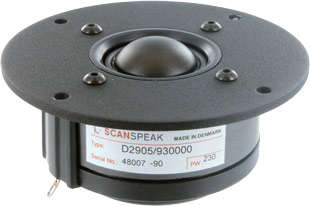open a bigger photo

recommended cabinet 1:
closed cabinet with 0 L volume
from 1275/805 Hz (-3dB/-8dB)

recommended cabinet 2:
0,01 L volume bass reflex cabinet
with 2cm-Rohr reflex tube, 20 cm long.
from 629/508 Hz (-3dB/-8dB).

D2905/930000       order no. ss-D2905-9300       EUR 114,97excl. VAT: € 96.61 / \$ 109.17
28 mm dome tweeter with fabric dome. The voice coil windings are immersed in magnetic fluid to increase short term power handling capacity, to reduce the compression and the Q factor..
• power handling (continuous/programme) = 150/460 W
• frequency range = 1000-33000 Hz
• resonance frequency fs = 650 Hz
• impedance R = 6 Ohm
• sound pressure level SPL = 90 dB (2,83V; 1m)
• DC resistance Re = 4,7 Ohm
• force factor BL = 3,5 N/A
• voice coil inductance L = 0,07 mH
• effective piston radiating area Sd = 8,5 cm2
• effective mechanical mass incl. air load mms = 0,45 g
• equivalent volume of compliance Vas = 0,01 l
• total Q factor Qts = 0,41 (Qms=0,94, Qes=0,72)
• voice coil diameter = 28 mm
• maximum peak linear excursion vibration xlin = +/- 0,4 mm
• mounting diameter d = 73,5 mm
• overall diameter d = 104,5 mm
• mounting depth (not countersunk) t = 43 mm
PDF data sheetopen a bigger photo

recommended cabinet 1:
closed cabinet with 0 L volume
from 1162/733 Hz (-3dB/-8dB)

recommended cabinet 2:
0,02 L volume bass reflex cabinet
with 2cm-Rohr reflex tube, 15 cm long.
from 588/468 Hz (-3dB/-8dB).

D2905/950000       order no. ss-D2905-9500       EUR 132,46excl. VAT: € 111.31 / \$ 125.78
28 mm dome tweeter with fabric dome. The voice coil windings are immersed in magnetic fluid to increase short term power handling capacity, to reduce the compression and the Q factor..
• power handling (continuous/programme) = 150/460 W
• frequency range = 1500-28000 Hz
• resonance frequency fs = 550 Hz
• impedance R = 6 Ohm
• sound pressure level SPL = 90 dB (2,83V; 1m)
• DC resistance Re = 4,7 Ohm
• force factor BL = 3,5 N/A
• voice coil inductance L = 0,07 mH
• effective piston radiating area Sd = 8,5 cm2
• effective mechanical mass incl. air load mms = 0,45 g
• equivalent volume of compliance Vas = 0,02 l
• total Q factor Qts = 0,38 (Qms=1,04, Qes=0,6)
• voice coil diameter = 28 mm
• maximum peak linear excursion vibration xlin = +/- 0,4 mm
• mounting diameter d = 73,5 mm
• overall diameter d = 104,3 mm
• mounting depth (not countersunk) t = 44 mm
PDF data sheet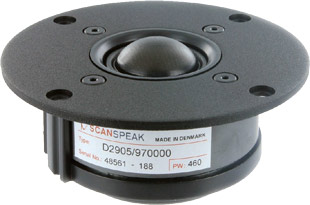open a bigger photo

recommended cabinet 1:
closed cabinet with 0,01 L volume
from 841/531 Hz (-3dB/-8dB)

recommended cabinet 2:
0,04 L volume bass reflex cabinet
with 2cm-Rohr reflex tube, 13 cm long.
from 394/329 Hz (-3dB/-8dB).

D2905/970000       order no. ss-D2905-9700       EUR 168,42excl. VAT: € 141.53 / \$ 159.93
28 mm dome tweeter with fabric dome.
• power handling (continuous/programme) = 225/460 W
• frequency range = 1000-28000 Hz
• resonance frequency fs = 500 Hz
• impedance R = 6 Ohm
• sound pressure level SPL = 89,5 dB (2,83V; 1m)
• DC resistance Re = 4,7 Ohm
• force factor BL = 3,5 N/A
• voice coil inductance L = 0,01 mH
• effective piston radiating area Sd = 8,5 cm2
• effective mechanical mass incl. air load mms = 0,45 g
• equivalent volume of compliance Vas = 0,02 l
• total Q factor Qts = 0,47 (Qms=3,45, Qes=0,54)
• voice coil diameter = 28 mm
• maximum peak linear excursion vibration xlin = +/- 0,4 mm
• mounting diameter d = 73,5 mm
• overall diameter d = 104,3 mm
• mounting depth (not countersunk) t = 44 mm
PDF data sheet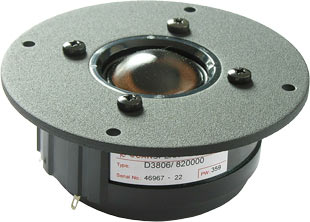open a bigger photo

recommended cabinet 1:
closed cabinet with 0,02 L volume
from 757/478 Hz (-3dB/-8dB)

recommended cabinet 2:
0,07 L volume bass reflex cabinet
with HP35 reflex tube, 21 cm long.
from 355/296 Hz (-3dB/-8dB).

D3806/820000       order no. ss-D3806-8200       EUR 181,20excl. VAT: € 152.27 / \$ 172.06
38 mm dome tweeter with fabric dome.
• power handling (continuous/programme) = 100 W
• frequency range = 1000-13,5000 Hz
• resonance frequency fs = 450 Hz
• impedance R = 6 Ohm
• sound pressure level SPL = 89 dB (2,83V; 1m)
• DC resistance Re = 5,7 Ohm
• force factor BL = 3,6 N/A
• voice coil inductance L = 0,04 mH
• effective piston radiating area Sd = 14 cm2
• effective mechanical mass incl. air load mms = 0,9 g
• equivalent volume of compliance Vas = 0,04 l
• total Q factor Qts = 0,48 (Qms=0,93, Qes=1)
• voice coil diameter = 38 mm
• maximum peak linear excursion vibration xlin = +/- 0,4 mm
• mounting diameter d = 97 mm
• overall diameter d = 125 mm
• mounting depth (not countersunk) t = 49 mm
PDF data sheet

## Discoveryopen a bigger photo

D2604/830000       order no. ss-D2604-830000       EUR 39,00excl. VAT: € 32.77 / \$ 37.03
26 mm dome tweeter with fabric dome.
• power handling (continuous/programme) = 100 W
• frequency range = 1000-24000 Hz
• resonance frequency fs = 630 Hz
• impedance R = 4 Ohm
• sound pressure level SPL = 92,1 dB (2,83V; 1m)
• DC resistance Re = 2,8 Ohm
• force factor BL = 2,2 N/A
• voice coil inductance L = 0,04 mH
• effective piston radiating area Sd = 8 cm2
• effective mechanical mass incl. air load mms = 0,42 g
• equivalent volume of compliance Vas = 0,01 l
• total Q factor Qts = 0,79 (Qms=3,46, Qes=1,02)
• voice coil diameter = 26 mm
• maximum peak linear excursion vibration xlin = +/- 0,3 mm
• mounting diameter d = 72 mm
• overall diameter d = 104 mm
• mounting depth (not countersunk) t = 32,9 mm
PDF data sheetopen a bigger photo

recommended cabinet 1:
closed cabinet with 0 L volume
from 877/553 Hz (-3dB/-8dB)

recommended cabinet 2:
0,02 L volume bass reflex cabinet
with 2cm-Rohr reflex tube, 30 cm long.
from 416/344 Hz (-3dB/-8dB).

R2604/832000       order no. ss-R2604-832000       EUR 52,00excl. VAT: € 43.70 / \$ 49.38
26 mm tweeter with fabric cone.
• power handling (continuous/programme) = 100 W
• frequency range = 1000-38000 Hz
• resonance frequency fs = 500 Hz
• impedance R = 4 Ohm
• sound pressure level SPL = 90 dB (2,83V; 1m)
• DC resistance Re = 2,9 Ohm
• force factor BL = 2,3 N/A
• voice coil inductance L = 0,02 mH
• effective piston radiating area Sd = 5,4 cm2
• effective mechanical mass incl. air load mms = 0,3 g
• equivalent volume of compliance Vas = 0,01 l
• total Q factor Qts = 0,44 (Qms=2,77, Qes=0,52)
• voice coil diameter = 26 mm
• maximum peak linear excursion vibration xlin = +/- 0,2 mm
• mounting diameter d = 72 mm
• overall diameter d = 104 mm
• mounting depth (not countersunk) t = 44,8 mm
PDF data sheet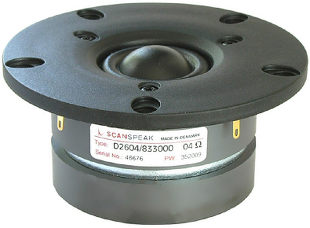open a bigger photo

recommended cabinet 1:
closed cabinet with 0,02 L volume
from 663/419 Hz (-3dB/-8dB)

recommended cabinet 2:
0,07 L volume bass reflex cabinet
with 2cm-Rohr reflex tube, 11 cm long.
from 295/258 Hz (-3dB/-8dB).

D2604/833000       order no. ss-D2604-833000       EUR 49,00excl. VAT: € 41.18 / \$ 46.53
26 mm dome tweeter with fabric dome.
• power handling (continuous/programme) = 100 W
• frequency range = 1000-30000 Hz
• resonance frequency fs = 475 Hz
• impedance R = 4 Ohm
• sound pressure level SPL = 93 dB (2,83V; 1m)
• DC resistance Re = 2,8 Ohm
• force factor BL = 2,2 N/A
• voice coil inductance L = 0,04 mH
• effective piston radiating area Sd = 8 cm2
• effective mechanical mass incl. air load mms = 0,42 g
• equivalent volume of compliance Vas = 0,02 l
• total Q factor Qts = 0,56 (Qms=2,55, Qes=0,71)
• voice coil diameter = 26 mm
• maximum peak linear excursion vibration xlin = +/- 0,3 mm
• mounting diameter d = 72 mm
• overall diameter d = 104 mm
• mounting depth (not countersunk) t = 60,2 mm
PDF data sheet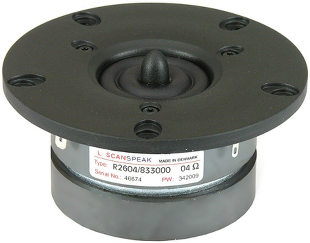open a bigger photo

recommended cabinet 1:
closed cabinet with 0,01 L volume
from 906/572 Hz (-3dB/-8dB)

recommended cabinet 2:
0,02 L volume bass reflex cabinet
with 2cm-Rohr reflex tube, 24 cm long.
from 455/364 Hz (-3dB/-8dB).

R2604/833000       order no. ss-R2604-833000       EUR 60,00excl. VAT: € 50.42 / \$ 56.97
26 mm tweeter with fabric cone.
• power handling (continuous/programme) = 100 W
• frequency range = 1000-38000 Hz
• resonance frequency fs = 440 Hz
• impedance R = 4 Ohm
• sound pressure level SPL = 92 dB (2,83V; 1m)
• DC resistance Re = 2,9 Ohm
• force factor BL = 2,3 N/A
• voice coil inductance L = 0,02 mH
• effective piston radiating area Sd = 5,4 cm2
• effective mechanical mass incl. air load mms = 0,3 g
• equivalent volume of compliance Vas = 0,02 l
• total Q factor Qts = 0,38 (Qms=2,18, Qes=0,46)
• voice coil diameter = 26 mm
• maximum peak linear excursion vibration xlin = +/- 0,2 mm
• mounting diameter d = 72 mm
• overall diameter d = 104 mm
• mounting depth (not countersunk) t = 60,1 mm
PDF data sheetopen a bigger photo

D2606/920000       order no. ss-D2606-920000       EUR 35,00excl. VAT: € 29.41 / \$ 33.24
26 mm dome tweeter with fabric dome.
• power handling (continuous/programme) = 100/200 W
• frequency range = 1000-22000 Hz
• resonance frequency fs = 1100 Hz
• impedance R = 6 Ohm
• sound pressure level SPL = 91,4 dB (2,83V; 1m)
• DC resistance Re = 4,6 Ohm
• force factor BL = 2,7 N/A
• voice coil inductance L = 0,04 mH
• effective piston radiating area Sd = 7,1 cm2
• effective mechanical mass incl. air load mms = 0,3 g
• equivalent volume of compliance Vas = 0,01 l
• total Q factor Qts = 0,84 (Qms=2,36, Qes=1,31)
• voice coil diameter = 26 mm
• maximum peak linear excursion vibration xlin = +/- 0,2 mm
• mounting diameter d = 72 mm
• overall diameter d = 104 mm
• mounting depth (not countersunk) t = 31,3 mm
PDF data sheetopen a bigger photo

recommended cabinet 1:
closed cabinet with 0,01 L volume
from 1208/762 Hz (-3dB/-8dB)

recommended cabinet 2:
0,03 L volume bass reflex cabinet
with 2cm-Rohr reflex tube, 7 cm long.
from 540/470 Hz (-3dB/-8dB).

D2606/922000       order no. ss-D2606-922000       EUR 49,00excl. VAT: € 41.18 / \$ 46.53
26 mm dome tweeter with fabric dome.
• power handling (continuous/programme) = 100/225 W
• frequency range = 1000-21000 Hz
• resonance frequency fs = 850 Hz
• impedance R = 6 Ohm
• sound pressure level SPL = 91,4 dB (2,83V; 1m)
• DC resistance Re = 4,6 Ohm
• force factor BL = 2,8 N/A
• voice coil inductance L = 0,04 mH
• effective piston radiating area Sd = 7,1 cm2
• effective mechanical mass incl. air load mms = 0,33 g
• equivalent volume of compliance Vas = 0,01 l
• total Q factor Qts = 0,57 (Qms=1,22, Qes=1,07)
• voice coil diameter = 26 mm
• maximum peak linear excursion vibration xlin = +/- 0,2 mm
• mounting diameter d = 72 mm
• overall diameter d = 104 mm
• mounting depth (not countersunk) t = 43,1 mm
PDF data sheet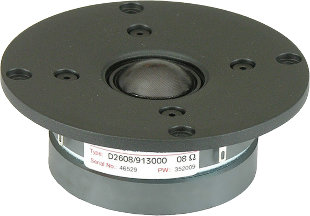open a bigger photo

recommended cabinet 1:
closed cabinet with 0 L volume
from 1989/1255 Hz (-3dB/-8dB)

recommended cabinet 2:
0,01 L volume bass reflex cabinet
with 2cm-Rohr reflex tube, 14 cm long.
from 1131/861 Hz (-3dB/-8dB).

D2608/913000       order no. ss-D2608-913000       EUR 78,00excl. VAT: € 65.55 / \$ 74.07
26 mm dome tweeter with fabric dome.
• power handling (continuous/programme) = 80/400 W
• frequency range = 1300-22000 Hz
• resonance frequency fs = 700 Hz
• impedance R = 8 Ohm
• sound pressure level SPL = 91,3 dB (2,83V; 1m)
• DC resistance Re = 5,6 Ohm
• force factor BL = 2,6 N/A
• voice coil inductance L = 0,04 mH
• effective piston radiating area Sd = 7 cm2
• effective mechanical mass incl. air load mms = 0,18 g
• equivalent volume of compliance Vas = 0,02 l
• total Q factor Qts = 0,29 (Qms=0,51, Qes=0,65)
• voice coil diameter = 26 mm
• maximum peak linear excursion vibration xlin = +/- 0,5 mm
• mounting diameter d = 72 mm
• overall diameter d = 104 mm
• mounting depth (not countersunk) t = 36,9 mm
PDF data sheet
 ASE Tweeter Full range Mids Woofer Subwoofer Car-HiFi no longer available
 Illuminator Revelator Classic Discovery
The cabinet volume calculations are executed considering an external resistance of 0.3 Ohm. We are using, if no other data is known or available, the information supplied by the manufacturer.

Please note: the frequency response doesn't say much about the cut-off frequency inside the cabinet! Therefore, we mostly give you 2 examples with dimensioning considerations and an indication, how low the loudspeaker will reproduce.

Home